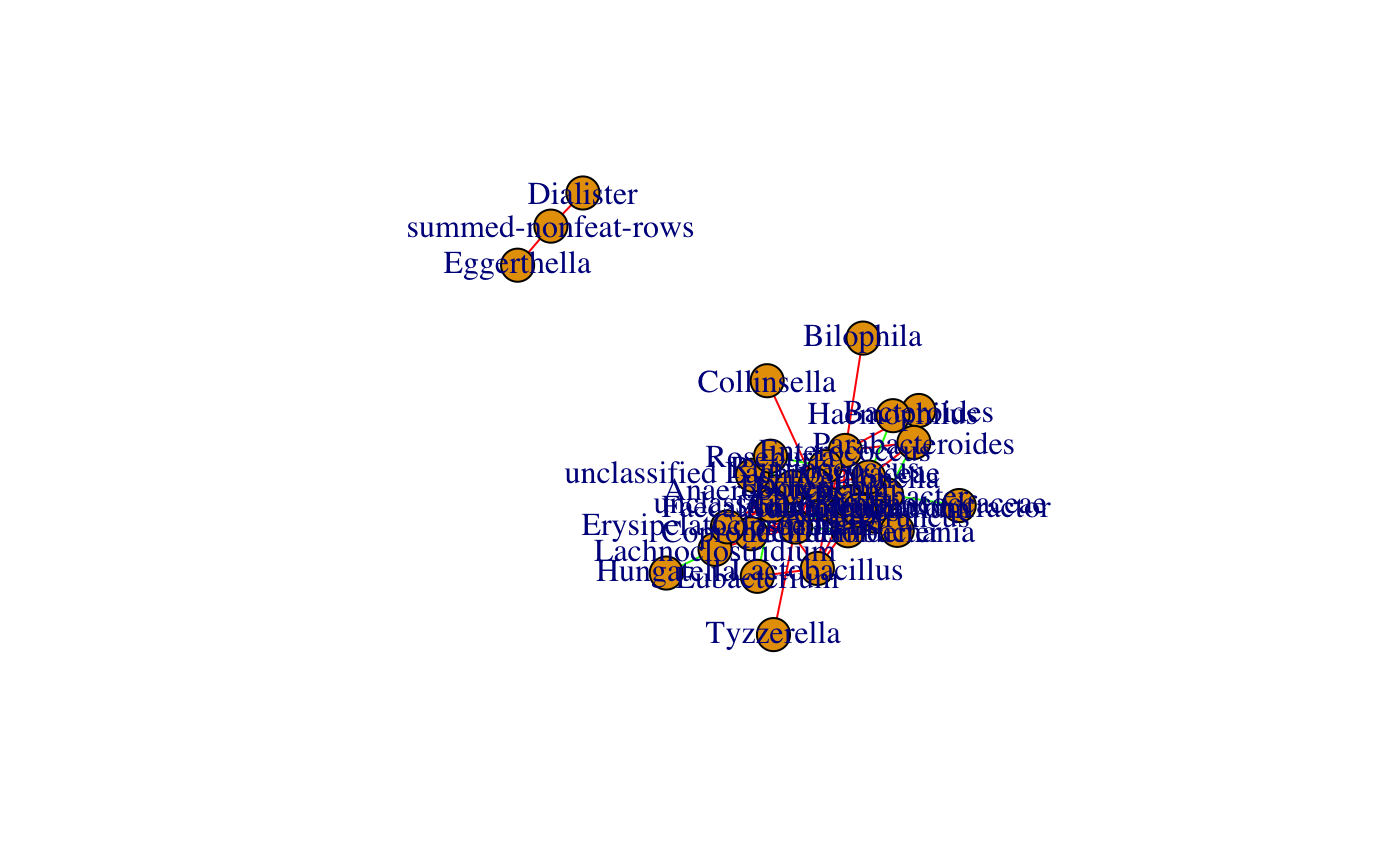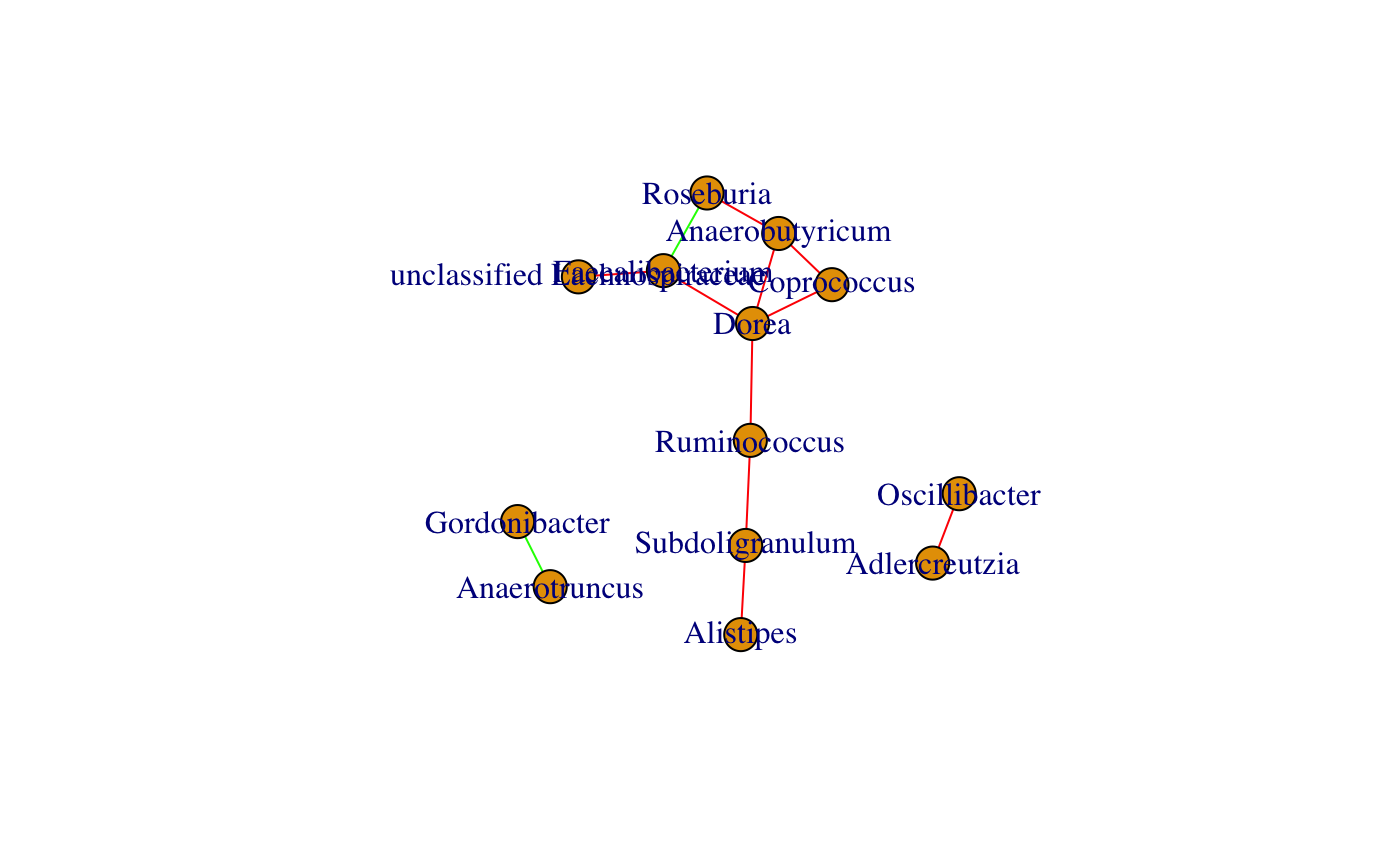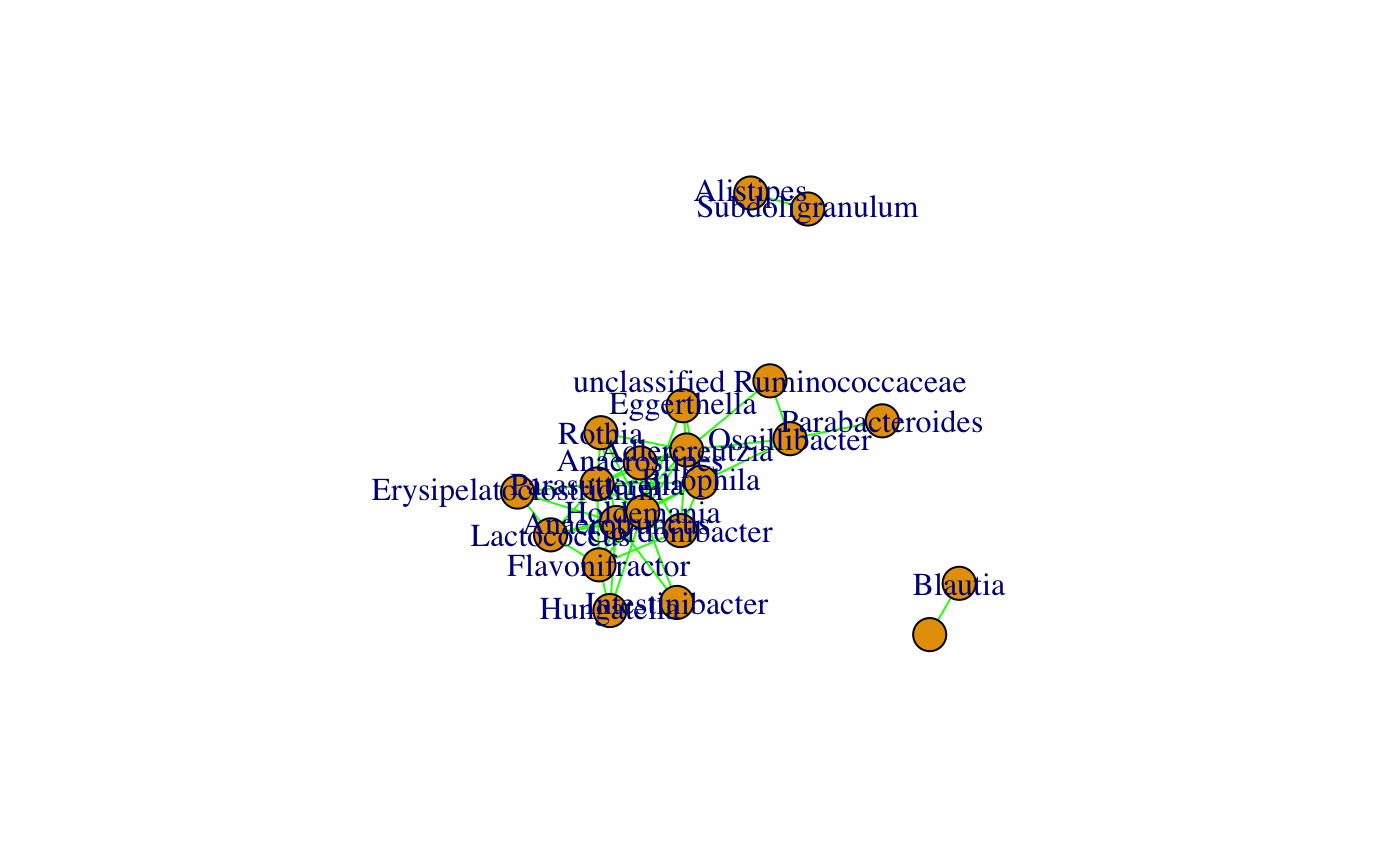Build a network using Pearson, Spearman, Euclidean, Kullback-Leibler and/or Bray-Curtis. The original CoNet implementation with extended functionality is available at: http://systemsbiology.vub.ac.be/conet. For export of data to the original CoNet, see exportToCoNet, for a generic network building function wrapping barebonesCoNet and other network inference methods, see buildNetwork.

barebonesCoNet(
abundances,
methods = c("spearman", "kld"),
T.up = NA,
T.down = NA,
method.num.T = 2,
pval.T = 0.05,
init.edge.num = max(2, round(sqrt(nrow(abundances)))),
min.occ = round(ncol(abundances)/3),
keep.filtered = TRUE,
norm = FALSE,
stand.rows = FALSE,
clr = FALSE,
pval.cor = FALSE,
permut = FALSE,
columnwise = FALSE,
renorm = FALSE,
permutandboot = FALSE,
iters = 100,
bh = TRUE,
pseudocount = 1e-11,
plot = FALSE,
verbose = FALSE
)

Arguments

abundances a matrix with taxa as rows and samples as columns an optional data frame with metadata items as columns, where samples are in the same order as in abundances and all items are numeric; a bipartite network will be computed network construction methods, values can be combinations of: "pearson", "spearman", "kld", "euclid" or "bray"; note that the latter two are not defined for negative abundance values upper threshold for scores (when more than one network construction method is provided, init.edge.num is given and/or p-values are computed, T.up is ignored) lower threshold for scores (when more than one network construction method is provided, init.edge.num is given and/or p-values are computed, T.down is ignored) threshold on method number (only used when more than one method is provided) threshold on p-value (only used when permut, permutandboot or pval.cor is true); if several methods are provided, only applied after merge the number of top and bottom initial edges (init.edge.num overrides T.up/T.down, set to NA to respect T.up/T.down for a single method) only keep rows with at least the given number of non-zero values (carried out before network construction) sum all filtered rows and add the sum vector as additional row normalize matrix (carrried out after filtering) standardize rows by dividing each entry by its corresponding row sum, applied after normalization apply CLR transform (after filtering and normalization); if true, stand.rows is ignored and keep.filtered is set to true; pseudocount is ignored for clr (clr with omit.zeros true) compute p-values of correlations with cor.test (only valid for correlations; takes precedence over permut and permutandboot with or without renorm) compute edge-specific p-values with a permutation test permute columns instead of rows for the permutation test use renormalization when computing permutation distribution (only applied to correlations; cannot be combined with metadata) compute p-values from both permutation (with or without renorm) and bootstrap distribution number of iterations for the permutation and bootstrap distributions multiple-test-correct using Benjamini-Hochberg; if several methods are provided bh is applied to merged p-value count added to zeros prior to taking logarithm (for KLD, p-value merge and significance) plot score or, if permut, permutandboot or pval.cor is true, p-value distribution, in both cases after thresholding print the number of positive and negative edges and, if permut, permutandboot or pval.cor is true, details of p-value computation

Value

igraph object with edge weights being either association strengths (single method), the number of supporting methods or, if permut, permutandboot or pval.cor is true, significances (-1*log10(pval))

Details

If renorm and permutandboot are both set to TRUE, p-value computation is equal to the ReBoot procedure implemented in CoNet. If more than one method is selected and p-value computation is enabled, p-values are merged with Fisher's method, multiple testing correction (if enabled) is applied on the merged p-value and the merged p-value is reported. If p-value computation is not enabled, the method number is reported as association strength. Edge signs (co-presence/mutual exclusion) are assigned using thresholds (T.up/T.down directly or indirectly via top/bottom initial edge number). Co-presence (high correlation/low dissimilarity) is encoded in green, mutual exclusion (low correlation/high dissimilarity) in red and sign conflicts (lack of agreement between methods) in gray. When metadata are provided, a bipartite network is computed. To circumvent bipartite network computation, metadata can be appended to abundances to form a single input matrix, but in this case, preprocessing on abundance data needs to be carried out before. When CLR transform is applied, the Euclidean distance is the recommended measure, which according to Gloor et al. (https://www.frontiersin.org/articles/10.3389/fmicb.2017.02224/full) is equivalent to the Aitchison distance after CLR.

Examples

data("ibd_taxa") data("ibd_lineages") ibd_genera=aggregateTaxa(ibd_taxa,lineages = ibd_lineages,taxon.level = "genus")
#>  "Number of higher-level taxa: 71" #>  71 7
# only keep significant ones among the top 50 positive and negative Spearman correlations plot(barebonesCoNet(ibd_genera,methods="spearman",init.edge.num=50,permutandboot=TRUE))
#>  "Network construction with method(s): spearman" #>  "Processing method spearman" #>  "Lower threshold for initial edge number (50): -0.269082821758457" #>  "Upper threshold for initial edge number (50): 0.476873894000414" #>  "Renormalisation is FALSE" #>  "P-values of correlations are computed with cor.test FALSE" #>  "Permutations and bootstraps are both computed TRUE" #>  "Associations computed for 46 taxa." #>  "Network has 99 edges."# only keep edges supported by both Bray Curtis and Spearman plot(barebonesCoNet(ibd_genera,methods=c("spearman","bray"),init.edge.num=50))
#>  "Network construction with method(s): spearman, bray" #>  "Upper and lower threshold are ignored when more than one method is selected." #>  "Processing method spearman" #>  "Lower threshold for initial edge number (50): -0.269082821758457" #>  "Upper threshold for initial edge number (50): 0.476873894000414" #>  "Renormalisation is FALSE" #>  "P-values of correlations are computed with cor.test FALSE" #>  "Permutations and bootstraps are both computed FALSE" #>  "Processing method bray" #>  "Lower threshold for initial edge number (50): 0.665322882108127" #>  "Upper threshold for initial edge number (50): 0.991535912658058" #>  "Renormalisation is FALSE" #>  "P-values of correlations are computed with cor.test FALSE" #>  "Permutations and bootstraps are both computed FALSE" #>  "Associations computed for 46 taxa." #>  "Merging methods..." #>  "Network has 12 edges."# keep significant Euclidean distance on CLR-transformed data (samples are completed to sum to one) g=rbind(ibd_genera,rep(1,ncol(ibd_genera))-colSums(ibd_genera)) plot(barebonesCoNet(g,clr=TRUE,methods="euclid",init.edge.num=50,permut=TRUE,columnwise=TRUE))
#>  "Network construction with method(s): euclid" #>  "Processing method euclid" #>  "Lower threshold for initial edge number (50): 27.941264917359" #>  "Upper threshold for initial edge number (50): 57.2944716021566" #>  "Renormalisation is FALSE" #>  "P-values of correlations are computed with cor.test FALSE" #>  "Permutations and bootstraps are both computed FALSE" #>  "Associations computed for 47 taxa." #>  "Network has 49 edges."